# What is the Point of a Pulley?

In its simplest implementation, a pulley simply turns a force in one direction into a force in another direction. This might be useful if it’s easier to apply a force in one direction than the other (e.g. to pull downwards rather than push upwards).

A pulley really comes into its own when it is combined with another pulley to create a system known as a block and tackle, allegedly invented by Archimedes in the third century BC.

The simplest block and tackle, the gun tackle, uses two pulleys, which are usually mounted above one another (for clarity, they are shown separated in the diagram below).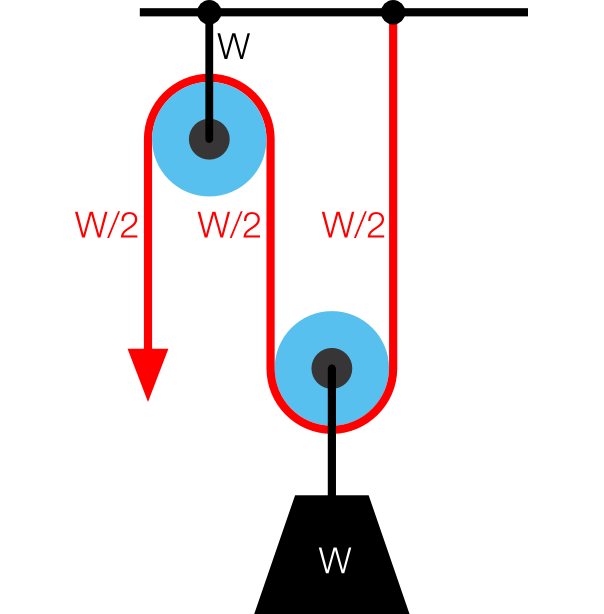The load (W) is shared between the tension in the rope and the mount that attaches the block and tackle to the ceiling, and thus you only have to pull with a force equal to half the weight in order to lift it. However, you will have to pull the rope twice as far and thus the conservation of energy is not violated (lifting a one hundred newton weight through one metre is the same amount of work as applying a fifty newton force over two metres).

The Luff tackle uses three pulleys in theory, but this is usually accomplished by running a rope over the top pulley twice. In this case the force required to lift a weight is reduced to one-third of its actual value, but again you have to pull further: three times the height required in this case.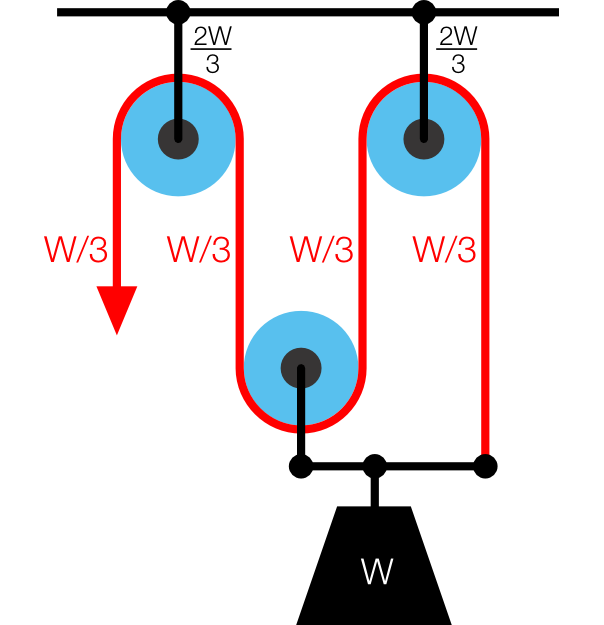A Luff tackle, shown separated.

The double tackle uses four pulleys, but similar to the Luff tackle, this is usually accomplished by running the rope twice over both pulleys.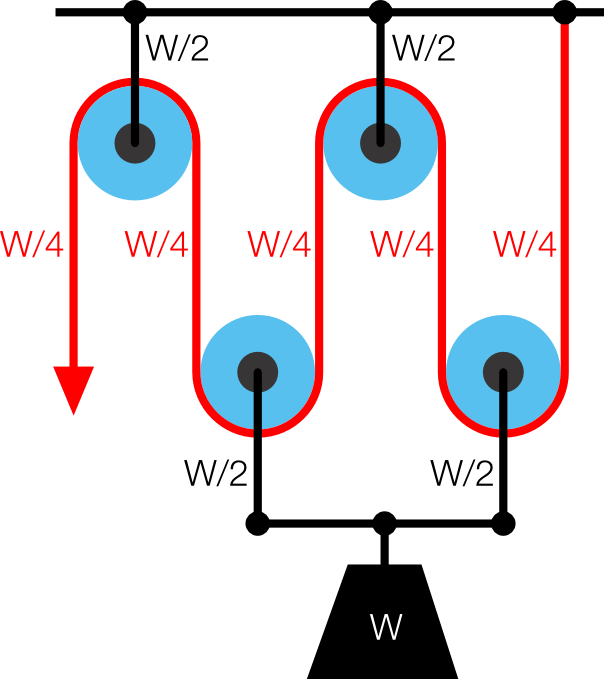In theory, this process can be continued indefinitely (one is reminded of Archimedes’ alleged remark: “Give me a place to stand and I will move the Earth“) but it quickly becomes impractical to do so, as the length of rope required, and the distance pulled through become unmanageable.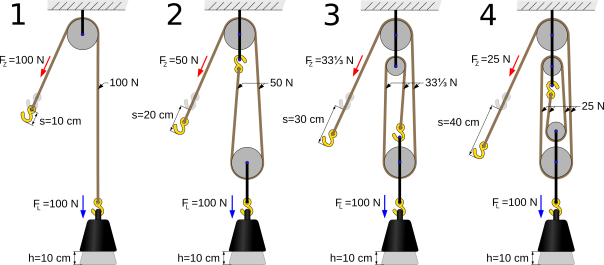Source [PDF]

A block and tackle can also be created using pulleys of different sizes.

# Lever Classes

The lever is one of the six simple machines.* It takes an input force and multiples it (by a factor known as the mechanical advantage) to create a larger output force.

Levers do not violate the conservation of energy because the input force has to be applied over a longer distance than the output force is applied over. (For example, a five newton force applied over one metre can produce a five hundred newton force over one centimetre; in both cases the work done is five joules.)

The operation of a lever is accomplished through the use of a rigid bar (the lever) and a fulcrum, or pivot. All levers can be placed into one of three classes depending of where the input force (red in the diagrams below), and output force (blue in the diagrams below) are applied relative to the fulcrum.

## Class 1

Examples: crowbar, scissors, pliers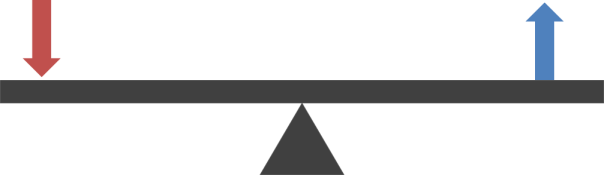A class one lever is the type most familiar to people, and is often compared to a seesaw. The output force and the fulcrum are usually very close together, giving a very large mechanical advantage, as in the case of a crowbar, which typically has a mechanical advantage of six or more.

## Class 2

Examples: wheelbarrow, nutcrackers, bottle opener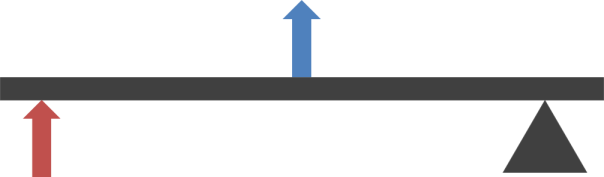Class 2 levers are less familiar; many people using  a class 2 lever will not have realised that are using a lever at all. A class 2 lever typically has a mechanical advantage of two or three.

## Class 3

Examples: tweezers, tongs, human jaw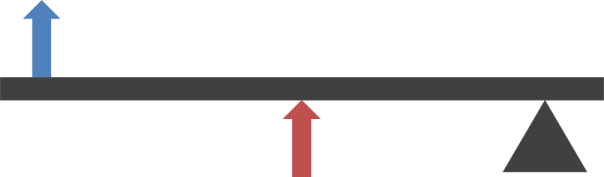Class 3 levers have a mechanical advantage of less than one, meaning that the output force is lower than the input force, and they are therefore not very common. They are only used when a force is required to be applied at a distance, as in the case of barbeque tongs or tweezers; or when using a class two lever would be impossible, as in the case of the human jaw (the jaw muscles would have to be in front of the teeth).

* The other five simple machines are the wheel & axle, the pulley, the inclined plane (i.e. the ramp), the wedge, and the screw.